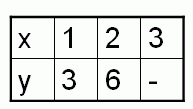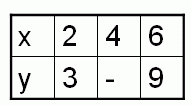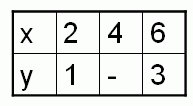kwizNET Subscribers, please login to turn off the Ads!
 Email us to get an instant 20% discount on highly effective K-12 Math & English kwizNET Programs!

#### Online Quiz (WorksheetABCD)

Questions Per Quiz = 2 4 6 8 10

### High School Mathematics9.3 Problems: Find the value of x and y

Example:
y = 5x, using this equation fill the following table.
x1-3
y-10-

Solution:
If x = 1 Ţ y = 5*1 = 5
When x = 1 Ţ y = 5
If y = 10 Ţ 10 = 5x
x = 10/5 = 2
When y = 10 Ţ x = 2
if x = 3 Ţ y = 5 * 3 = 15
When x = 3 Ţ y = 15.

Directions: Fill the below tables.

 Q 1: If y = x/3, then find the missing value.9687 Q 2: If y = 3x, then find the missing value.9876 Q 3: If y = 3x/2, then find the missing value.8567 Q 4: If y = x/2, then find the missing value.6824 Question 5: This question is available to subscribers only! Question 6: This question is available to subscribers only!

#### Subscription to kwizNET Learning System offers the following benefits:

• Unrestricted access to grade appropriate lessons, quizzes, & printable worksheets
• Instant scoring of online quizzes
• Progress tracking and award certificates to keep your student motivated
• Unlimited practice with auto-generated 'WIZ MATH' quizzes
• Child-friendly website with no advertisements
• Choice of Math, English, Science, & Social Studies Curriculums
• Excellent value for K-12 and ACT, SAT, & TOEFL Test Preparation
• Get discount offers by sending an email to discounts@kwiznet.com

 Quiz Timer